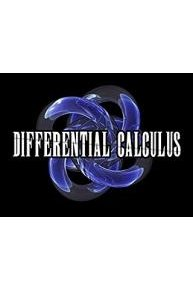# Calculus I (Differential Calculus)

Calculus I is designed primarily for those students planning to pursue programs in engineering, mathematics, computer science, and physical sciences. This course includes topics of differential and integral calculus of a single variable.

Math Fortress
1 Season, 9 Episodes
iEpisodes

## Calculus I (Differential Calculus) Full Episode Guide

• This is the third and final video on the quotient rule. This video goes over 4 examples illustrating how to find the derivative of a function by using a set of values and by using the graphs of functions.

• This video continuous going over more challenging example that require the use of the quotient. This video goes over 7 examples. 3 of these examples involves finding an expression for the derivative in terms of general functions.

• This video will teach you how to find the derivative of functions formed by a quotient of functions by using the quotient rule and going over 3 examples.

• This video continues going over how to find the derivative of functions formed by products of functions by using the product rule. This videos goes over 2 examples illustrating how to find the derivative of functions by using the graphs of these functions.

• This video continues going over how to find the derivative of functions formed by products of functions by using the product rule. This videos goes over 5 examples illustrating how to find the derivative of functions by writing a general expression and using values that are provided to find the derivative at a given value of x.

• This video will teach you how to find the derivative of functions formed by products of functions by using the product rule. An example involving the product of three functions is also illustrated.

• This video will teach how to rewrite common functional expressions into a "derivative friendly" form. A good understanding of intermediate algebra is required to succeed in any calculus class.

• This video will teach you the basics of calculating the derivative of simple polynomials and exponential functions using the constant multiple, sum and difference rule.

• This video will teach you the basics of calculating the derivative of simple polynomials and exponential functions.